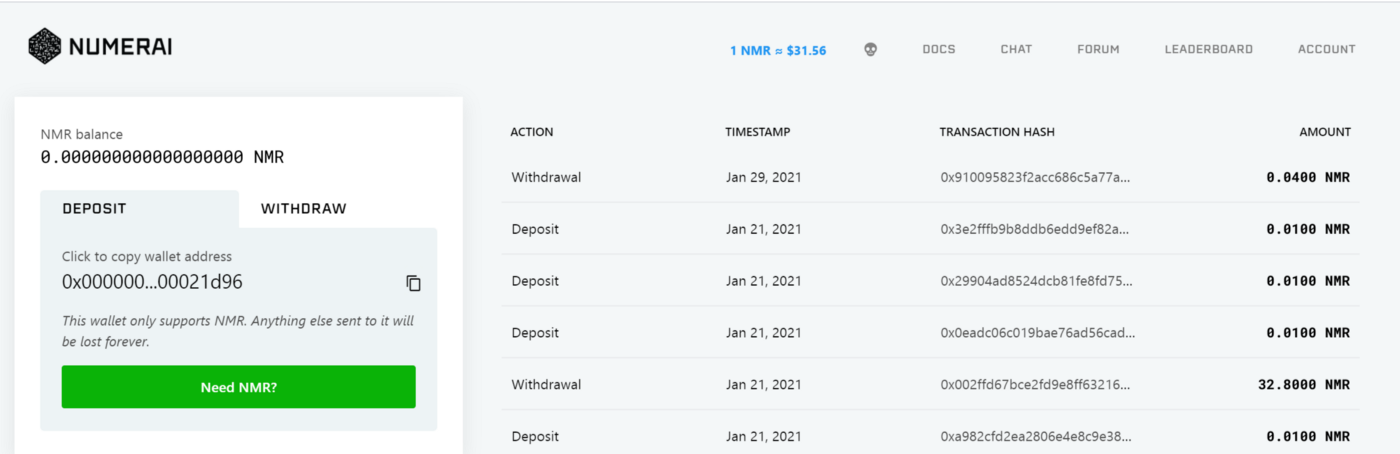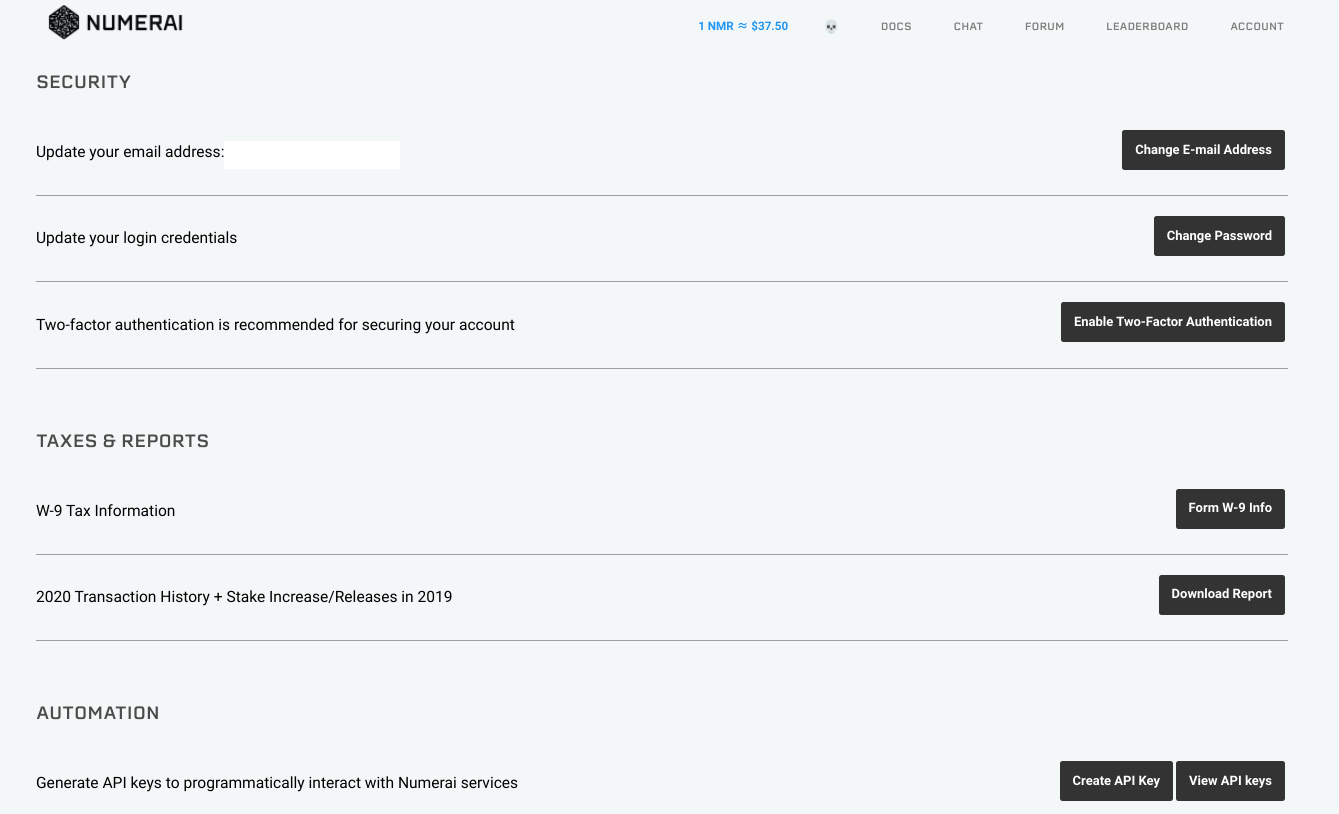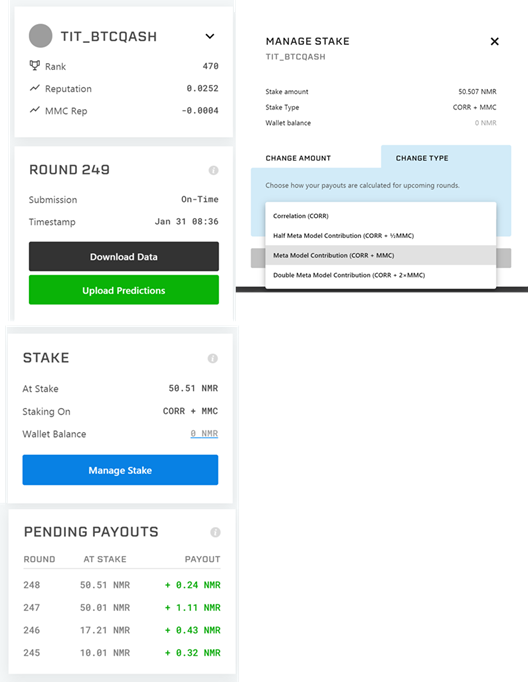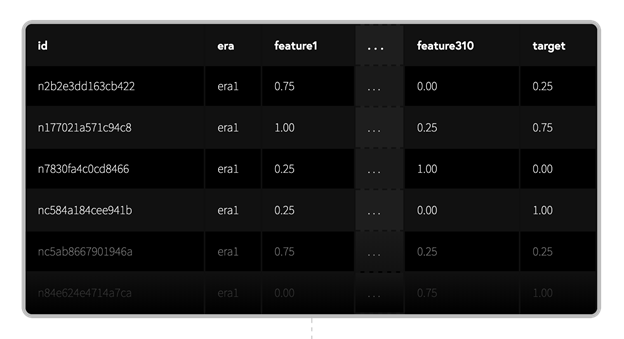## はじめに

2015年に設立されたNumeraiは、毎週データサイエンスのトーナメントを開催しています。世界中のデータサイエンティストが、難読化されたデータを無料でダウンロードし、株式市場の予測モデルを構築することでトーナメントに参加できます。ダウンロードできるデータは難読化されたいるため、金融の知識がなくても参加することができます。 参加者は、自分の予測に賭け金をかけることでトーナメントに参加できます。良い予測を提出すると、より多くの仮想通貨（NMR）を得ることができ、悪い予測を提出すると、NMRを没収されます。 Numeraiは、メタモデルと呼ばれる参加者のモデルを統合したモデルを構築し、得られたデータを自社のグローバル・エクイティ・ヘッジファンドの取引に反映させています。 参加者は、賭け金の有無にかかわらずトーナメントに参加することができますが、NumeraiはNMRがステークされたモデルのみを使用します。参加者は自分でデータを用意する必要はありませんが、チームから与えられたデータを最適化し、自分の予測を提出することが求められます。

## 目次

1. 1.
Numeraiのホームページと用語集の読み方
2. 2.
Numeraiに予測を提出する方法
3. 3.
モデル診断の読み方
4. 4.
Numeraiとコミュニティに関連する便利なリンク

## 1. Numeraiのホームページと用語集の読み方

NumeraiのホームページのURLは、https://numer.ai/tournament です。 Numeraire（NMR）トークン: NumeraireはERC-20ベースのトークンで、Numeraiへのステーキングに使用されます。 NMRは、Numeraiのトーナメントに参加したり、Numeraiに技術的な貢献をすることで獲得できます。NMRトークンは、Numeraiが構築されているErasure Protocolで使用することができます。現在、Erasureプロトコル上のアプリは、Numerai、Numerai Signals、Erasure Bayですが、プロトコルは誰でも構築できるようになっています。 Corr： 提出された予測データとターゲットとの相関係数を表します。 MMC： メタモデル貢献度（MMC）は、モデルの予測がNumeraiのメタモデルにとってどれだけ価値があるかを示します。非常に独創的な予測は、独創的でない予測よりも高いMMCを持ちます。MMCに関する詳細はこちら FNC: Feature neutral correlation （FNC）は、提出した予測データをNumeraiのすべての特徴量に対して中和した後の、ターゲットとの相関係数を表します。 Corr / MMC / FNC Rep: Rep（評判）は、過去20ラウンドにおけるその指標の加重平均であり、リーダーボードでユーザーをランク付けするために使用されるものです。Repについての詳細はこちら ステーク 自分のモデルの予測にどれだけ自信があるかを示すために、NMRをステーク（預入れ＋ロック）をします。良い予測を提出した場合、NMRを得ることができます。一方、悪い予測を提出した場合はNMRが没収されます。賭け金の最小値は3NMRです。ステークせずに参加して、自分のモデルがどのようなパフォーマンスを示すかを知ってから、ステークするかどうかを決めることができます。 ペイアウト 賭けたNMRに応じて受け取る報酬。毎週（1ラウンドは4週間）、賭け金の最大25％を獲得または没収されます。ステーキングとペイアウトについての詳細はこちらをご覧ください。

# ホームページ上部DOCS: ルール、FAQ、新規ユーザーセクションを含むNumeraiのドキュメントへのリンクです。 CHAT: 告知、一般的な議論、データサイエンスなどのチャンネルを含むチャットスペースです。NumeraiチームはRocketChatに積極的に参加しており、プロフィールには`team`タグが付いています。チームメンバーは主に太平洋時間で活動しています。参加はこちらから。 FORUM: Numerai、データサイエンス、ステイク戦略などに関連する議論のためのスペースです。主にコミュニティ内での情報共有を目的としています。リンクはこちらです。 LEADERBOARD： Numeraiに投稿された予測結果のランキングです。ステーク量、Rep、MMCなどでソート可能です。 アカウント: 「ウォレット」「モデル」「設定」「ログアウト」の4つのリンクがあります。 ・ウォレット：NMRトークンの入金と出金が行えます。 入金(deposit):ウォレットに登録されているアドレスにNMRトークンを送ることで入金できます。このアドレスに他のトークンを送ることはできません。 出金(withdraw):NMRトークンを自分のNumeraiウォレット以外のアドレスに出金することができます。出金を依頼する前に、出金先の外部アドレスがNMR受け取りに適していることを確認してください。間違ったアドレスに送ったNMRは回収できません。モデル このページでは、Numeraiに投稿するモデルを追加／削除できます。モデルの追加／削除をするには、「新しいモデルの追加」／「既存のアカウントの吸収」を押してください。 複数モデルのアカウントやアカウントの吸収についてはこちらをご覧ください。# ホームページ下部

モデル情報： モデル（今回はTIT_BTCQASH）のランキングやRep、MMC Repなどの情報を掲載しています。↓のボタンでモデルを切り替えることができます。 データ情報: 最新ラウンドのデータダウンロードリンクと、予測結果のアップロードリンクです。 ステーク: NMRのステーク量を調整できます。ステーク方法には、CorrやCorr＋MMCなどの種類があります。例えば、Corrではターゲットとの相関関係のみにNMRを賭けることができ、Corr + MMCではターゲットとの相関関係とMMCにNMRを賭けることができます。 Pending Payouts: 各ラウンドの予想ペイアウト（払い出し）の表です。# 2. Numeraiに予測を提出する方法

すぐにでもNumeraiに投稿したいという方は、大会参加者のkatsu1110さんCarlo LepelaarsさんのKaggle Notebooksがとても参考になります。 今回の記事では、上記の記事や公式のサンプルモデルから一歩踏み込んだ説明（コードでどこを改善するかなど）をします。本説明により提出プロセスがわかりやすくなり、大会の出場者数が増えることを願っています。 今回紹介したコードは、Google Colab上で実行できます。Runボタンを押すと投稿ファイルが作成されますので、ぜひ使ってみてください。 Colabへのリンク

## i) Numeraiデータセットの構造

データセットはダウンロードリンクからダウンロードできます。ファイルを解凍すると、numerai_training_data.csv、numerai_tournament_data.csvなどのファイルがあることがわかります。numerai_training_data.csvはトレーニングデータを格納したcsvファイル、numerai_tournament_data.csvは検証用データを格納したcsvファイルです。id: 暗号化された株のラベル era: データが収集された期間のことです。eraが同じであれば、同じ期間にデータが収集されたことを意味します。 data_type: データの種類。trainはトレーニング用のデータ、validationは検証用のデータ、testはテスト用のデータ、liveは現在のラウンドのデータです。 feature: ビン化した特徴量。特徴量は0,0.25,0.5,0.75,1の5段階にビン化されています。また、特徴量は、次のようなラベルの付いたグループに分かれています。 "feature_intelligence"、"feature_wisdom"、"feature_charisma"、"feature_dexterity"、"feature_strength"、"feature_constitution" target: ビン化された教師データ。また、ターゲットも0,0.25,0.5,0.75,1の5段階にビン化されています。numerai_training_data.csvではターゲットデータが与えられていますが、numerai_tournament_data.csvのテストデータとライブデータではNaNとなっています。

## ii）データ提出までの流れ

1. 1.
データ読み込み
2. 2.
特徴量エンジニアリング
3. 3.
機械学習
4. 4.
モデルの強さについて
5. 5.
予測結果が書き込まれたcsvファイルの作成
6. 6.
中和の方法

## 2A.データ読み込み

1
!pip install numerapi
2
import numerapi
3
NAPI = numerapi.NumerAPI(verbosity="info")
4
import numpy as np
5
import random as rn
6
import pandas as pd
7
import seaborn as sns
8
import lightgbm as lgb
9
import matplotlib.pyplot as plt
10
from scipy.stats import spearmanr, pearsonr
11
from sklearn.metrics import mean_absolute_error
12
import os
13
14
DIR = "/kaggle/working"
15
16
"""
17
18
:param directory: The path to the directory where the data needs to be saved
19
"""
20
current_round = NAPI.get_current_round()
21
if os.path.isdir(f'{directory}/numerai_dataset_{current_round}/'):
22
23
else:
24
25
26
27
def load_data(directory: str, reduce_memory: bool=True) -> tuple:
28
"""
29
Get data for current round
30
:param directory: The path to the directory where the data needs to be saved
31
:return: A tuple containing the datasets
32
"""
33
34
full_path = f'{directory}/numerai_dataset_{NAPI.get_current_round()}/'
35
train_path = full_path + 'numerai_training_data.csv'
36
test_path = full_path + 'numerai_tournament_data.csv'
37
38
39
# Reduce all features to 32-bit floats
40
if reduce_memory:
41
num_features = [f for f in train.columns if f.startswith("feature")]
42
train[num_features] = train[num_features].astype(np.float32)
43
test[num_features] = test[num_features].astype(np.float32)
44
val = test[test['data_type'] == 'validation']
45
test = test[test['data_type'] != 'validation']
46
return train, val, test
47
48
49
train, val, test = load_data(DIR, reduce_memory=True)
Copied!

## 2B.特徴量エンジニアリング

Numeraiデータセットの特徴量は互いに相関が低く、特徴量エンジニアリングを行わなくてもある程度の結果が得られます。 まず、訓練データを見てみると、大まかに6種類に分かれていることがわかります。 （"feature_intelligence"、"feature_wisdom"、"feature_charisma"、"feature_dexterity"、"feature_strength"、"feature_constitution "） Carlo Lepelaars氏の論文からコードを引用しましたが、これらの特徴の平均値、偏差値、歪度などは有用な特徴です。 したがって、train = get_group_stats (train)を呼び出して、これらの特徴をtrainデータに追加します。
1
def get_group_stats(df: pd.DataFrame) -> pd.DataFrame:
2
for group in ["intelligence", "wisdom", "charisma", "dexterity", "strength", "constitution"]:
3
cols = [col for col in df.columns if group in col]
4
df[f"feature_{group}_mean"] = df[cols].mean(axis=1)
5
df[f"feature_{group}_std"] = df[cols].std(axis=1)
6
df[f"feature_{group}_skew"] = df[cols].skew(axis=1)
7
return df
8
train = get_group_stats(train)
9
val = get_group_stats(val)
10
test = get_group_stats(test)
Copied!
1
from sklearn import preprocessing
2
ft_corr_list=['feature_dexterity7', 'feature_charisma18', 'feature_charisma63', 'feature_dexterity14']#Please try other features!
3
interactions = preprocessing.PolynomialFeatures(degree=2, interaction_only=True, include_bias=False)
4
5
interactions.fit(train[ft_corr_list], train["target"])
6
7
X_train_interact = pd.DataFrame(interactions.transform(train[ft_corr_list]))
8
X_best_val_inter =pd.DataFrame(interactions.transform(val[ft_corr_list]))
9
X_best_test_inter =pd.DataFrame(interactions.transform(test[ft_corr_list]))
10
11
train=pd.concat([train,X_train_interact],axis=1)
12
13
val=val.reset_index().drop(columns='index')
14
val=pd.concat([val,X_best_val_inter],axis=1)
15
16
test=test.reset_index().drop(columns='index')
17
test=pd.concat([test,X_best_test_inter],axis=1)
Copied!
Kaggleで使われているような特徴量エンジニアリングがNumeraiでもそのまま使えるので、train、val、testのデータを加工ことで、良いCorrやSharpe ratioが得られると思います。Numeraiで良い結果を得るために必要な作業の一つが特徴量エンジニアリングであることに間違いはありません。

## 2C.機械学習

Numeraiデータセットに機械学習を適用する際に考慮しなければならないのは i) どのような機械学習手法を使用するか（LightGBM、XGBoost、ニューラルネットワークなど) ii) どのようなハイパーパラメータを使用するか iii) 予測結果をスタックするかどうか などです。 今回は、計算時間を考慮してLightGBMを使用します。訓練データ以外のid、era、datatypeは機械学習には必要ありません。残ったfeature ○○を説明変数として、targetを教師データとして学習します。学習したデータを用いて、valに含まれるValidationデータとLiveデータについても予測データを作成します。 i)～iii)を考慮すれば、Corr等の値が改善されるので、この部分もやりこみ要素の要素の一つです。
1
feature_list = train.columns.drop(['id','era','data_type','target'])
2
dtrain = lgb.Dataset(train[feature_list].fillna(0), label=train["target"])
3
dvalid = lgb.Dataset(val[feature_list].fillna(0), label=val["target"])
4
5
best_config ={"objective":"regression", "num_leaves":31,"learning_rate":0.01,"n_estimators":2000,"max_depth":5,"metric":"mse","verbosity": 10, "random_state": 0}
6
7
model = lgb.train(best_config, dtrain)
8
9
train.loc[:, "prediction"] = model.predict(train[feature_list])
10
11
val.loc[:,"prediction"]=val["target"]
12
val.loc[:,"prediction"] = model.predict(val[feature_list])
Copied!

## 2D.モデルの強さについて

spearman, payout, numerai_sharpe, maeを計算して、Validationデータのモデルの強さを推定することができます。 spearman,payout,numerai_sharpeは大きいほど良いです。 この中でも特にspearmanの値が大きい（0.025以上が目安）と良いモデルであるとみなせます。 (※Corrだけに注目すると、いろいろな問題が発生する可能性があります。Numeraiに詳しい方とは意見が分かれるところだと思いますが、初めて予測結果を提出する方向けの記事なので、このように表現させてください) なお、用語の説明は以下の通りです。 spearman： Correlationの平均値。高ければ高いほど良い(参考は0.022～0.04) ペイアウト 平均リターン numerai_sharpe： 平均リターンを標準偏差で割った比率。高ければ高いほど良い（目安は1以上） mae： 平均絶対誤差
1
def sharpe_ratio(corrs: pd.Series) -> np.float32:
2
"""
3
Calculate the Sharpe ratio for Numerai by using grouped per-era data
4
5
:param corrs: A Pandas Series containing the Spearman correlations for each era
6
:return: A float denoting the Sharpe ratio of your predictions.
7
"""
8
return corrs.mean() / corrs.std()
9
10
11
def evaluate(df: pd.DataFrame) -> tuple:
12
"""
13
Evaluate and display relevant metrics for Numerai
14
15
:param df: A Pandas DataFrame containing the columns "era", "target" and a column for predictions
16
:param pred_col: The column where the predictions are stored
17
:return: A tuple of float containing the metrics
18
"""
19
def _score(sub_df: pd.DataFrame) -> np.float32:
20
"""Calculates Spearman correlation"""
21
return spearmanr(sub_df["target"], sub_df["prediction"])
22
23
# Calculate metrics
24
corrs = df.groupby("era").apply(_score)
25
print(corrs)
26
payout_raw = (corrs / 0.2).clip(-1, 1)
27
spearman = round(corrs.mean(), 4)
28
29
payout = round(payout_raw.mean(), 4)
30
numerai_sharpe = round(sharpe_ratio(corrs), 4)
31
mae = mean_absolute_error(df["target"], df["prediction"]).round(4)
32
33
# Display metrics
34
print(f"Spearman Correlation: {spearman}")
35
print(f"Average Payout: {payout}")
36
print(f"Sharpe Ratio: {numerai_sharpe}")
37
print(f"Mean Absolute Error (MAE): {mae}")
38
return spearman, payout, numerai_sharpe, mae
39
40
feature_spearman_val = [spearmanr(val["prediction"], val[f]) for f in feature_list]
41
feature_exposure_val = np.std(feature_spearman_val).round(4)
42
spearman, payout, numerai_sharpe, mae = evaluate(val)
Copied!

## 2E.予測結果が書き込まれたcsvファイルの準備

1
test.loc[:, "prediction"] =0
2
test.loc[:, "prediction"] = model.predict(test[feature_list])
3
test[['id', "prediction"]].to_csv("submission_test.csv", index=False)
4
5
val[['id', "prediction"]].to_csv("submission_val.csv", index=False)
6
7
test=0
8
val=0
9
10
directory = "/kaggle/working"
11
full_path = f'{directory}/numerai_dataset_{NAPI.get_current_round()}/'
12
13
test_path = full_path + 'numerai_tournament_data.csv'
14
15
16
tournament_data_id=tournament_data['id']
17
tournament_data_id2=tournament_data['feature_dexterity7']
18
tournament_data_id=pd.concat([tournament_data_id,tournament_data_id2],axis=1)
19
20
21
22
23
test_val_concat=pd.concat([val[['id', "prediction"]],test[['id', "prediction"]]],axis=0).set_index('id')
24
tournament_data_id=tournament_data_id.set_index('id')
25
26
conc_submit=pd.concat([tournament_data_id,test_val_concat],axis=1).drop(columns='feature_dexterity7').reset_index()
27
conc_submit=conc_submit.rename(columns={'index': 'id'})
28
conc_submit.to_csv("submission_file"+".csv", index=False)
Copied!

## 2F.中和の方法

1
def neutralize(series,by, proportion):
2
scores = series.values.reshape(-1, 1)
3
exposures = by.values.reshape(-1, 1)
4
exposures = np.hstack((exposures, np.array([np.mean(series)] * len(exposures)).reshape(-1, 1)))
5
correction = proportion * (exposures.dot(np.linalg.lstsq(exposures, scores)))
6
corrected_scores = scores - correction
7
neutralized = pd.Series(corrected_scores.ravel(), index=series.index)
8
return neutralized
9
10
11
12
13
neut=pd.DataFrame({'prediction':neutralize(neut['prediction'],by['prediction'], 0.3)})
14
15
conc=pd.concat([by.drop(columns="prediction"),neut],axis=1)
16
conc.to_csv("neutralized_submission_file.csv", index=False)#submission file
Copied!

# 4.新規参加者向けのTips

### Numeraiとコミュニティに関連する便利なリンク

• RocketChat #newUsers チャンネルに参加することでさらなるヒントやサポートを得られます！
• Slack に参加することで、日本人参加者とコミュニケーションがとれます！
• データ分析、ヒント、チュートリアルに関する投稿は、Numeraiフォーラムをチェックしてください。